UGC NET CS 2010 June-Paper-2

 Question 1
“x1 is a clone of x” means x1 is identical to x in terms of the physical attributes namely, height, weight and complexion. Given, height, weight and complexion only form a complete set of attributes for an entity, cloning is an equivalence relation. What is your impression about this statement ?
 A The statement is true B The statement is false C The truth value of the statement cannot be computed D None of these
Engineering-Mathematics       Set-Theory
Question 1 Explanation:
The given statement says that the Relation R contains x and x1 which are identical. Which means (x,x1) are same as (x,x).
We know that " a relation of (x,x) type is an equivalence as it satisfies Reflexive, symmetric, transitive.
The answer would be option A, it is equivalence.
 Question 2
‘R is a robot of M’ means R can perform some of the tasks that otherwise M would do and R is unable to do anything else. Which of the following is the most appropriate representation to model this situation ?
 ABC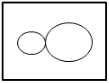D None of these
Question 2 Explanation:
Given data,
-- R is a robot of M
Step-1: Constraint is the R can perform some tasks of M. It means M can do all tasks.
But sometimes R can’t do which can do by M.
Step-2: The above constraint clearly representing that M is superset and R is subset of M.
We can represent this in venn diagrams areQuestion 3
“My Lafter Machin(MLM) recognizes the following strings :
(i) a
(ii) aba
(iii) abaabaaba
(iv) abaabaabaabaabaabaabaabaaba
Using this as an information, how would you compare the following regular expressions ?
(i) (aba)3x
(ii) a.(baa)3x–1. ba
(iii) ab.(aab).3x–1.a
 A (ii) and (iii) are same, (i) is different. B (ii) and (iii) are not same. C (i), (ii) and (iii) are different. D (i), (ii) and (iii) are same.
Theory-of-Computation       Regular-Expression
Question 3 Explanation:
m is generating single "a".
If you observe all of them is generating (aba)x
Note: This question is ambiguous, the wordings are not very clear.
 Question 4
S1 : I teach algorithms and maths.
S2 : My professor teaches maths, electronics and computer science.
S3 : I have a student of maths.
S4 : Algorithm is a part of computer science.
S5 : Maths students know computer science.
What would be the chromatic number of a graph, vertices of which are the actors/entities that are involved in the sentences S1 to S5 and edges-to represent the associations/relationships amongst the entities/actors as expressed in the sentences S1 to S5 above ?
 A 2 B 3 C 4 D None of these
Engineering-Mathematics       Graph-Theory
 Question 5
Four your ATM debit card, you have a 4-decimal-digit personal secret code. In the absence of any clue, a brute-force attack takes time-‘t’ to crack the code on an ATM terminal. Therefore ‘t’ is the secure-time for a customer to report in case the card is misplaced. Your Bank has decided to facilitate an increased secure-time. Out of the following, which option should provide the largest rise in the value of ‘t’ ?
 A Instead of 4-decimal-digits, maintain the personal secret code in 4-hexadecimal-digits. B Instead of 4-decimal digits, maintain a 5-decimal-digit personal secret code. C Reduce the processing speed of the ATM terminals to the half of their current speed. D None of the above provides any improvement.
Question 5 Explanation:
→ To get the password of 4 digits possible number digit would be 104 which has brute-force attack takes time-‘t’.
Therefore ‘t’ is the secure-time for a customer to Increase the secure more time we have to increase the size of the password of 5 digits or more.
 Question 6
The logic expression for the output of the circuit shown in the figure isA A’C’ + B’C’ + CD B AC’ + BC’ +C’D C ABC + C’D’ D A’B’ + B’C’ + C’D’ E None of the above
Digital-Logic-Design       Logic-Gates
Question 6 Explanation:
[((A+B)'+c)'+(c+d)']'
=((A+B)'+C)(C+D)
=(A'B'+C)(C+D)
=A'B'C+C+A'B'D+CD
=(A'B'+1+D)+A'B'D
=A'B'D
Note: They given wrong options.
 Question 7
Advantage of synchronous sequential circuits over asynchronous ones is
 A faster operation B ease of avoiding problems due to hazard C lower hardware requirement D better noise immunity E None of the above
Digital-Logic-Design       Sequential-Circuits
Question 7 Explanation:Note: Excluded for evaluation.
 Question 8
What is the transitive voltage for the voltage input of a CMOS operating from 10V supply ?
 A 1V B 2V C 5V D 10 V
 Question 9
What is decimal equivalent of BCD 11011.1100 ?
 A 22.0 B 22.2 C 20.2 D 21.2 E None of the above
Digital-Logic-Design       Number-Systems
Question 9 Explanation:
The question is not properly framed.
In the question it is mentioned that 11011.1100 is BCD.
We know that BCD code is different from Binary number system.
Each of the decimal digit has a 4-bit binary code or in other words every block of 4 bits has a corresponding decimal digit.
0000- 0
0001- 1
:
:
1001- 9
1010 - 1111 are not valid codes.
If the given code 11011.1100 is BCD then divide it into blocks of 4 bits.
0001 1011. 1100 (Note: Appending zeros on the left side will not change value.)
But 1011 is not a valid BCD code.
 Question 10
The function represented by the k- map given below isA A ⋅ B B AB + BC + CA C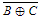D A . B . C
Digital-Logic-Design       K-Map
Question 10 Explanation:Note: Above options are wrong.
 Question 11
The statement
printf(“%d”,10 ? 0 ? 5 : 1 : 12);
will print
 A 10 B 0 C 12 D 1
Programming       Operator
Question 11 Explanation:
We can write above statement into (10 ? (0 ? 5 : 1) : 12)
Step-1: (0 ? 5 : 1) → It will give result 1 because the condition is FALSE because given value is 0. If nonzero given in condition, it will print TRUE value.
Step-2: (10 ? 1 : 12) → Here, given condition is nonzero. So, it will print TRUE value. The TRUE value is 1.
Note: Ternary operators(?:) evaluation starts from innermost conditions first.
 Question 12
What will be the output of the following c-code ?
void main ( )
{
char *P = "ayqm" ;
char c;
c = ++*p ;
printf ("%c", c);
}
 A a B c C b D q
Programming       Operator
Question 12 Explanation:
Pointer "p" will point to the string "ayqm",
*p means the first character of the string which is "a"
++*p means an increment of "a" which is nothing but "b"
printf ("%c", c); means the character "b" will be printed as output.
Note: They given syntax error in program.
 Question 13
Member of a class specified as _______ are accessible only to method of the class.
 A private B public C protected D derive
Programming-in-c++       Declaration
Question 13 Explanation:
→ Member of a class specified as private are accessible only to method of the class.
→ Private is default for a member of a class if no access specifier is used for it.
→ When we pass an argument by call by value a copy of argument is made into the formal parameter of the subroutine and changes made on parameters of subroutine have no effect on original argument, they remain the same.
 Question 14
Match the following :A (a)-1, (b)-5, (c)-4, (d)-2, (e)-3 B (a)-1, (b)-5, (c)-2, (d)-3, (e)-4 C (a)-5, (b)-1, (c)-2, (d)-3, (e)-4 D (a)-5, (b)-4, (c)-3, (d)-1, (e)-2
Database-Management-System       Normalization
Question 14 Explanation:
Garbage collection in→ Java
Nameless object→ Within a statement
Template support→ Generic programming
A forward reference→ defines a class
Derived class inherits from base class→ Member function
 Question 15
The data type created by the data abstraction process is called
 A class B structure C abstract data type D user defined data type
Programming-in-c++       Abstraction
Question 15 Explanation:
→ The data type created by the data abstraction process is called abstract data type(ADT).
→ ADT is a class of objects whose logical behavior is defined by a set of values and a set of operations.
 Question 16
An entity instance is a single occurrence of an _______.
 A entity type B relationship type C entity and relationship type D None of these
Database-Management-System       ER-Model
Question 16 Explanation:
→ Entity type is the heading or schema and entity instance is the value of entity type at a particular instance.
Example:
PERSON(Age, Name, Address) is the Entity Type and PERSON( 28, Anand, Hyderabad) is the entity instance i.e. value of PERSON at a particular instance.
 Question 17
Generalization is _______ process.
 A top-down B bottom up C both (A) & (B) D None of these
Question 17 Explanation:
Generalization can be defined as the process of extracting the common characteristics from two or more classes and combining them into a generalized superclass. Generalization is a Bottom Up approach.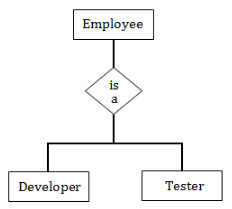Question 18
Match the following :A I-(a), II-(c), III-(b), IV-(d) B I-(d), II-(a), III-(b), IV-(c) C I-(c), II-(d), III-(a), IV-(b) D I-(d), II-(b), III-(a), IV-(C) E I-(c), II-(a), III-(b), IV-(d)
Database-Management-System       Normalization
Question 18 Explanation:
→ 2NF ensures that the relation contains no partial dependency. A relation is in 2NF if it is in 1NF and have no partial dependency.
→ 3NF eliminates transitive dependency from the relation. A relation can be in 3NF if it is in 2NF and have no transitive dependency.
→ 4NF eliminates multivalued dependency from the relation. A relation is in 4NF if it is in BCNF and have no multivalued dependency.
→ 5NF ensures that the relation contains no join dependency. A relation is in 5NF if it is 4NF and ave no join dependency.
Note: Given question is wrong. We are added correct extra option. Excluded for correction.
 Question 19
Which data management language component enabled the DBA to define the schema components ?
 A DML B Sub-schema DLL C Schema DLL D All of these
Database-Management-System       SQL
Question 19 Explanation:
Database task Group(DBTG) specified three distinct data management language components to produce the required standardization
1. Schema DDL: The schema DDL enabled the DBA to define the schema components.
2. Subschema DDL: It allowed the application programs to define the database components that will be used by the program
3. DML: It enables the manipulation of the database contents.
 Question 20
The PROJECT Command will create new table that has
 A more fields than the original table B more rows than original table C both (A) & (B) D none of these
Database-Management-System       SQL
Question 20 Explanation:
Project command is used to select a column of a relation based on the condition given in the query.
Option(A) is incorrect because the maximum number of columns Project command can select is equal to the total number of columns in the given relation.
Option(B) is incorrect because project command is related to the columns of a relation, it is not related to the selection of rows in the table. The result of Project command contain same number of rows as that of given relation.
 Question 21
If we have six stack operations pushing and popping each of A, B and C such that push(A) must occur before push(B) which must occur before push(C), then A, C, B is a possible order for the pop operations,since this could be our sequence : push(A), pop(A), push(B), push(C), pop(C), pop(B). Which one of the following orders could not be the order the pop operations are run, if we are to satisfy the requirements described above ?
 A ABC B CBA C BAC D CAB
Data-Structures       Queues-and-Stacks
Question 21 Explanation:Option-A: According to constraint,
Push(A) then Pop(A)
Push(B) then Pop(B)
Push(C) then Pop(C)
Then the possible pop sequence is ABC. So, it is TRUE
Option-B: According to given constraint, Push(A),Push(B) and Push(C) then Pop(C),
Pop(B) and Pop(A). The possible Pop sequence is CBA. So, it is TRUE
Option-C: According to given constraint, Push(A) and Push(B) then Pop(B) and Pop(A)
then push(C) and Pop(C). The possible Pop sequence is BAC. So, it is TRUE
Option-D: This is not possible.
 Question 22
What is the most appropriate data structure to implement a priority queue ?
 A Heap B Circular array C Linked list D Binary tree
Data-Structures       Queues-and-Stacks
Question 22 Explanation:
→ Priority queue is an abstract data type which is like a regular queue or stack data structure, but where additionally each element has a "priority" associated with it.
→ In a priority queue, an element with high priority is served before an element with low priority.
→ In some implementations, if two elements have the same priority, they are served according to the order in which they were enqueued, while in other implementations, ordering of elements with the same priority is undefined.
→ While priority queues are often implemented with heaps, they are conceptually distinct from heaps. A priority queue is an abstract concept like "a list" or "a map".
→ just as a list can be implemented with a linked list or an array, a priority queue can be implemented with a heap or a variety of other methods such as an unordered array.
 Question 23
In a complete binary tree of n nodes, how far are the two most distant nodes? Assume each edge in the path counts as !
 A About log2n B About 2 log2n C About n log2n D About 2n
Data-Structures       Binary-Trees
Question 23 Explanation:
According to binary two most distinct nodes are in
level-1: 1 and 2
level-2: 3 and 6.
[Note: Assume that root starts from level-0]The height of the binary tree is O(log2n). As per the given constraint, we have to calculate individually of left farthest node and right farthest node height.
=log2n*log2n
=2*log2n or (log2n)2
 Question 24
A chained hash table has an array size of 100. What is the maximum number of entries that can be placed in the table ?
 A 100 B 200 C 10000 D There is no upper limit
Data-Structures       Hashing
Question 24 Explanation:
Here, two things we have to remember
1. They given chained hash. It is like linked list.
2. Maximum number of entries that can be placed in the table.
In chained hash, we adding new element when collisions are happened.
 Question 25
In a B tree of order 5, the following keys are inserted as follows : 7, 8, 1, 4, 13, 20, 2, 6 and 5 How many elements are present in the root of the tree ?
 A 1 B 2 C 3 D 4
Database-Management-System       B-and-B+-Trees
Question 25 Explanation:
Since order of B-tree is 5.
⇒ Then maximum no. of key that can be present in a node =5-1=4keys
⇒ Minimum no. keys that can be present in a node =⌈52⌉-1=2 keys
⇒ Now 7, 8, 1, 4 are inserted in a node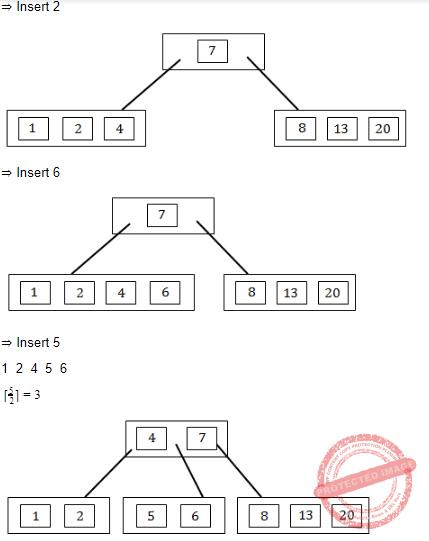Question 26
The ______ field is the SNMP PDV reports an error in a response message.
 A error index B error status C set request D agent index
Question 26 Explanation:
Simple network management protocol protocol data units(SNMP PDU)
It has many number of fields.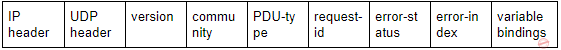Error status: Indicates one of a number of errors and error types. Only the response operation sets this field. Other operations set this field to zero.
Error index: Associates an error with a particular object instance. Only the response operation sets this field. Other operations set this field to zero.
 Question 27
What does the URL need to access documents ?
I. Path name
II. Host name
III. DNS
IV. Retrieval method
V. Server port number
 A I, II, III B I, III, V C I, II, IV D III, IV, V
Computer-Networks       URL
Question 27 Explanation:
The URL need to access documents are Path name, DNS and Server port number.
 Question 28
End-to-End connectivity is provided from Last-to-Last in
 A Network layer B Session layer C Transport layer D Data link layer
Computer-Networks       OSI-TCP-layers
Question 28 Explanation:
→ Data link layer-Process to Process connectivity
→ Network layer-Host to Host connectivity
→ Transport layer-End to End connectivity
→ Presentation layer-Encryption and Decryption
 Question 29
What services does the internet layer provide ?
1. Quality of service
2. Routing
4. Connection oriented delivery
5. Framing bits
 A 1, 2, 3 B 2, 3, 4 C 1, 3, 4, 5 D 2, 3, 4, 5
Computer-Networks       TCP/IP-Layers
Question 29 Explanation:
→ Internet Protocol provides Quality of Service, Routing, Addressing. It does not provides Connection oriented service and Framing is the responsibility of data link layer.
 Question 30
What is the maximum operating rate of a wireless LAN using infrared communication?
 A 1 mbps B 2 mbps C 5 mbps D 11mbps
Computer-Networks       LAN
Question 30 Explanation:
The maximum operating rate of a wireless LAN using infrared communication is 1 Mbps.
 Question 31
In an absolute loading scheme, which loader function is accomplished by a loader ?
Question 31 Explanation:
Loader loads the executable code into memory, program and data stack are created, register gets initialized.
 Question 32
Which of the following expression is represented by the parse tree ?A (A + B) * C B A + * BC C A + B * C D A * C + B
Compiler-Design       Parsers
Question 32 Explanation:
Parse tree is always following inorder traversal. It visit left,root and right.Question 33
Consider the following left associative operators in decreasing order of precedence :
– subtraction (highest precedence)
* multiplication
\$ exponentiation (lowest precedence)
What is the result of the following expression ?
3 – 2 * 4 \$ | * 2**3
 A – 61 B 64 C 512 D 4096
Compiler-Design       Compiler-Design
Question 33 Explanation:
Actually they are given in wrong order.
But according to given question, we are giving precedence is
(((3 – 2) *) 4 \$ | * (2**3))
Step-1: 3-2=1
Step-2: 1*4=4
Step-3: 2*3=6
Step-4: 46=4096
Note: When we are assuming ** is single(*) and there is no |* are useless symbols.
 Question 34
Which of the following is the most general phase structured grammar ?
 A Regular B Context-sensitive C Context free D None of the above
Theory-of-Computation       Languages-and-Grammars
Question 34 Explanation:
→ Phrase structure grammars are also known as constituency grammars. The defining trait of phrase structure grammars is thus their adherence to the constituency relation, as opposed to the dependency relation of dependency grammars.
→ Most general phase structured grammar is context sensitive grammar.
 Question 35
Which of the following is used for grouping of characters into tokens(in a computer)?
 A A parser B Code optimizer C Code generator D Scanner
Compiler-Design       Phases-of-Compilers
Question 35 Explanation:
Lexical analysis(or Scanner) used for grouping of characters into tokens.
 Question 36
Match the following :A (a)-3, (b)-4, (c)-2, (d)-1 B (a)-4, (b)-3, (c)-2, (d)-1 C (a)-2, (b)-4, (c)-1, (d)-3 D (a)-1, (b)-4, (c)-3, (d)-2
Operating-Systems       Process-Scheduling
Question 36 Explanation:
Disk Scheduling→ SCAN
Batch Processing→ FIFO
Time Sharing→ Round-robin
Interrupt Processing→ LIFO
 Question 37
________ synchronizes critical resources to prevent deadlock.
 A P-operator B V-operator C Semaphore D Swapping
Question 37 Explanation:
Semaphore synchronizes critical resources to prevent deadlock.
 Question 38
_______ is one of preemptive scheduling algorithm.
 A RR B SSN C SSF D Priority based
Operating-Systems       Process-Scheduling
Question 38 Explanation:
Round Robin(RR) is one of preemptive scheduling algorithm. Priority based scheduling is non preemptive.
 Question 39
In order to allow only one process to enter its critical section, binary semaphore are initialized to
 A 0 B 1 C 2 D 3
Operating-Systems       Semaphores
Question 39 Explanation:
→ In order to allow only one process to enter its critical section, binary semaphore are initialized to 1.
→ It will change its state after enters into critical section. It will be 0.
 Question 40
Remote Computing Service involves the use of time sharing and _______.
 A multi-processing B interactive processing C batch processing D real-time processing
Operating-Systems       Types-of-Operating-System
Question 40 Explanation:
Remote Computing Service involves the use of time sharing and batch processing.
 Question 41
Software engineering primarily aims on
 A reliable software B cost effective software C reliable and cost effective software D none of the above
Software-Engineering       Software-design
Question 41 Explanation:
Software engineering primarily aims on reliable and cost effective software.
 Question 42
Top-down design does not require
 A stepwise refinement B loop invariants C flow charting D modularity
Question 42 Explanation:
→ Top-down design does not require loop invariants.
→ A loop invariant is a condition that is necessarily true immediately before and immediately after each iteration of a loop.
 Question 43
Which model is simplest model in Software Development ?
 A Waterfall model B Prototyping C Iterative D None of these
Software-Engineering       Types-of-Models
Question 43 Explanation:
Waterfall model is simplest and oldest software development life cycle model.
 Question 44
Design phase will usually be
 A top-down B bottom-up C random D centre fringing
Software-Engineering       Software-design
Question 44 Explanation:
Design phase will usually be top-down approach.
 Question 45
Applications-software
 A is used to control the operating system B includes programs designed to help programmers C performs a specific task for computer users D all of the above
Software-Engineering       Software-design
Question 45 Explanation:
Applications software performs a specific task for computer users.
 Question 46
The cost of the network is usually determined by
 A time complexity B switching complexity C circuit complexity D none of these
Question 46 Explanation:
The cost of the network is usually determined by switching complexity.
 Question 47
A leased special high-speed connection from the local telephone carrier for business users that transmits at 1.544 mbps is known as________ carrier.
 A T1 B T2 C T3 D T4
Question 47 Explanation:Question 48
CDMA Cell uses ________ carriers of 1.25 MHz.
 A 9 B 18 C 22 D 64
Question 48 Explanation:
A CDMA system has 1.25 MHz wideband carriers
– Carrier bandwidth in AMPS is 30 kHz
– Carrier bandwidth in GSM is 200 kHz
– Carrier bandwidth in IS-95 is 1.23 MHz
– 1.25MHz with guard band
→ The recommended guard band between the CDMA carrier band edge and an AMPS or TDMA carrier is 270 KHz ⇒ 9 AMPS channels of 30 kHz.
 Question 49
At any given time Parallel Virtual Machine (PVM) has ________ send buffer and ________ receive buffer.
 A one-one B one-two C two-two D two-one
Question 49 Explanation:
Parallel Virtual Machine(PVM): It Is a software package that allows a heterogeneous collection of workstations (host pool) to function as a single high performance parallel machine (virtual).
→ At any given time Parallel Virtual Machine (PVM) has one send buffer and two receive buffer.
 Question 50
Data Mining uses ________,________ and ________ to build effective predictive model.
(i) Data set
(ii) Information set
(iii) Input set
(iv) Process set
(v) Output set
(vi) Test set
 A (i), (ii) and (iv) B (ii), (iv) and (v) C (i), (v) and (vi) D (ii), (iii) and (v)
Question 50 Explanation:
Data Mining uses data set, information set and process set to build effective predictive model.
There are 50 questions to complete.
PHP Code Snippets Powered By : XYZScripts.com